# Coordinate Geometry Notes | Study Mathematics (Maths) Class 11 - JEE

## JEE: Coordinate Geometry Notes | Study Mathematics (Maths) Class 11 - JEE

The document Coordinate Geometry Notes | Study Mathematics (Maths) Class 11 - JEE is a part of the JEE Course Mathematics (Maths) Class 11.
All you need of JEE at this link: JEE

A. COORDINATE GEOMETRY

Coordinate Geometry is the unification of algebra and geometry in which algebra is used in the study of geometrical relations and geometrical figures are represented by means of equations. The most popular coordinate system is the rectangular Cartesian system. Coordinates of a point are the real variables associated in an order to describe its location in space. Here we consider the space to be two-dimensional.

Through a point O, referred to as the origin, we take two mutually perpendicular lines XOX' and YOY' and call them x and y axes respectively. The position of a point is completely determined with reference to these axes by means of an ordered pair of real numbers (x, y) called the coordinates of P where | x | and | y | are the distances of the point P from the y-axis and the x-axis respectively, x is called the xcoordinate or the abscissa of P and y is called the y-coordinate or the ordinate of the point P.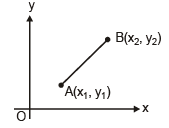(1) Distance between two points :

(a) Let A and B be two given points, whose coordinates are given by A(x1, y1) and B(x2 , y2) respectively.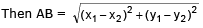(b) Distance of (x1, y1) from origin :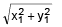Note :- If two vertex A(x1, y1), B(x2, y2) are given then third vertex of equilateral triangle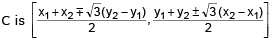(2) Section formula :

Coordinates of the point P dividing the join of two points A(x1, y1) and B(x2, y2) internally in the given  ratio λ1 : λ2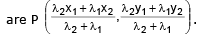Coordinates of the point P dividing the join of two points A(x1, y1) and B(x2, y2) externally in the ratio λ1 : λ2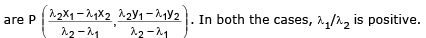(3) Special points in a triangle with co-ordinates :

(a) Centroid (G)

Definition : The point of concurrence of the medi ans of a triangle is called the centroid of the triangle.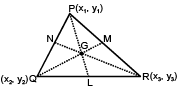(i) G divides median into 2 : 1.

(ii) G always lies inside the triangle.

(iii) Co-ordinates of G is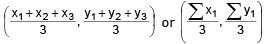(b) Incentre (I) :

Definition : The point of concurrency of the internal bisectors of the angles of a triangle is called the incentre of the triangle.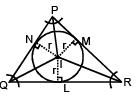(i) I always lies inside the triangle.

(ii) Internal angle bisector divides the base in the ratio of adjacent sides.

(iii) Co-ordinates of I is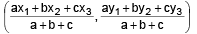where a, b, c are the lengths of the sides of the Δ

(c) Ex-centres (I1, I2, I3) :

Definition : The centre of the escribed circle which is opposite to vertices.

To get I1 (or I2 or I3) replace a by –a (b by –b or c by –c) in formula of coordinate of I

(d) Circumcentre (C) :

Definition : The point of concurrency of the perpendicular bisectors of the sides of a triangle is called circumcentre of the triangle.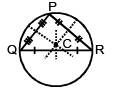(i)    For acute angle Δ ⇒  lies inside

(ii)   For obtuse angle Δ ⇒  lies outside

(iii)  For right angle Δ ⇒  Mid point of hypotenuse

(iv)  Co-ordinates of circumcentre is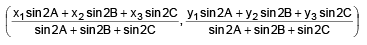(e) Orthocentre (O) :

Definition : The point of concurrency of the altitudes of a triangle is called the orthocentre of the triangle.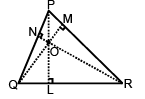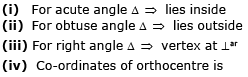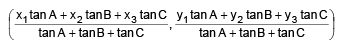Notes :

(i) In any triangle O, G, C are collinear.

(ii) In any triangle G divides the line joining O & C in ratio 2 : 1.

(iii) In an equilateral triangle O, G, C, I are coincident.

(iv) In an isosceles triangle O, G, C, I are collinear.

(f) Harmonic Conjugate : If P is a point that divides AB internally in the ratio m1: m2 and Q is another point which divides AB externally in the same ratio m1 : m2, then the point P and Q are said to be Harmonic conjugate to each other with respect to A and B.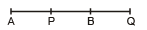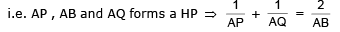Note :- Internal and External angle bisector of an angle divides the base harmonically.

Ex. If midpoints of the sides of a triangle are (0, 4), (6, 4) and (6, 0), then find the vertices of triangle, centroid and circumcentre of triangle.

Sol. Let points A(x1, y1), B(x2, y2) and C(x3, y3) be vertices of ΔABC.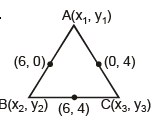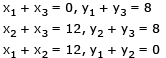Solving we get A(0, 0), B (12, 0) and C(0, 8).

Hence ΔABC is right angled triangle ∠A = π/2

Circumcentre is midpoint of hypotenuse which is (6, 4) itself and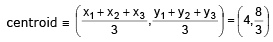Ex.2 Prove that the incentre of the triangle whose vertices are given by A(x1, y1), B(x2, y2), C(x3, y3) is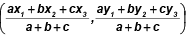where a, b, and c are the sides opposite to the angles  A, B and C respectively.

Sol.

By geometry, we know that BD/DC = AB/AC

If the length of the sides AB, BC and AC are c, a and b respectively, then  BD/DC = AB/AC  = c/b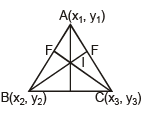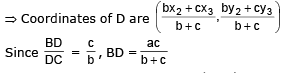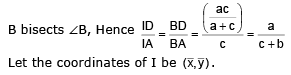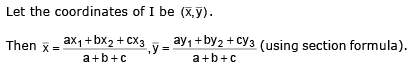The document Coordinate Geometry Notes | Study Mathematics (Maths) Class 11 - JEE is a part of the JEE Course Mathematics (Maths) Class 11.
All you need of JEE at this link: JEEUse Code STAYHOME200 and get INR 200 additional OFF

## Mathematics (Maths) Class 11

154 videos|171 docs|132 tests

Track your progress, build streaks, highlight & save important lessons and more!

,

,

,

,

,

,

,

,

,

,

,

,

,

,

,

,

,

,

,

,

,

;